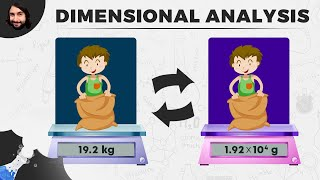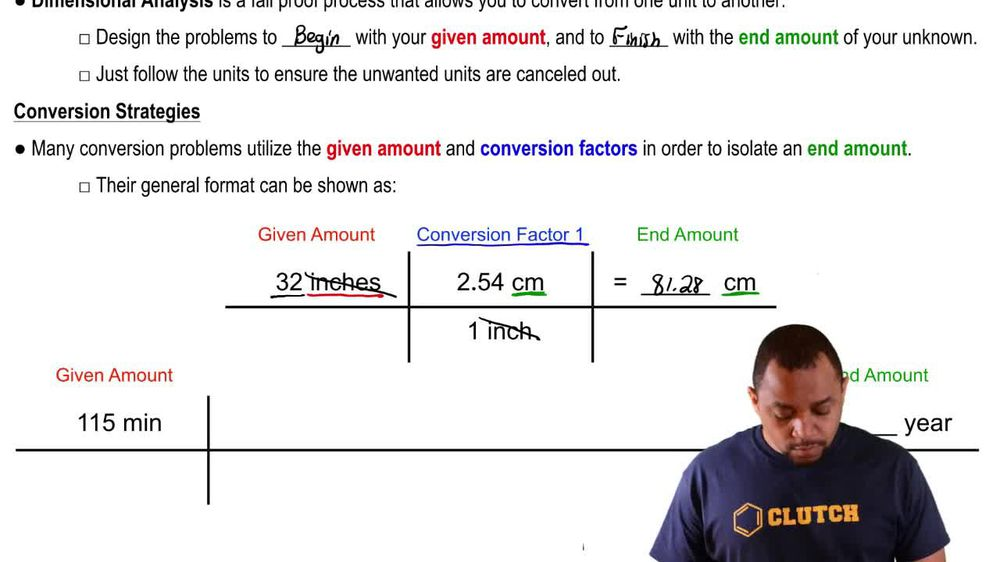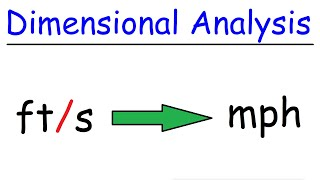Start typing, then use the up and down arrows to select an option from the list.
1. 1. Intro to General Chemistry2. Dimensional Analysis
Problem

# The density of polystyrene, a plastic commonly used to make CD cases and transparent cups, is 0.037 lbs/in3. Calculate the density in units of g/cm3.

Relevant Solution1m
Play a video:
Hi everyone today, we have a question asking us to calculate the density of nickel in pounds per feet cubed. If it is 8.902 kg per meter cube. Our first step here is going to be to remember our conversion factors. So we know that one kg Equals £2.205. And we know that one m Equals 3.281 ft. And now we're going to put this in an equation. So we're going to start with our eight . and that is kilograms per meter cube. And now we're going to multiply that by our conversion factor. So £2. over one kg. So are kilograms here are going to cancel out. And then we're going to multiply that by one m Over 3.281 ft. And this whole part of the equation is cute. So that's going to take away our meters cubed And that is going to equal 0. six pounds per feet cubed. And that is our final answer. Thank you for watching. Bye.